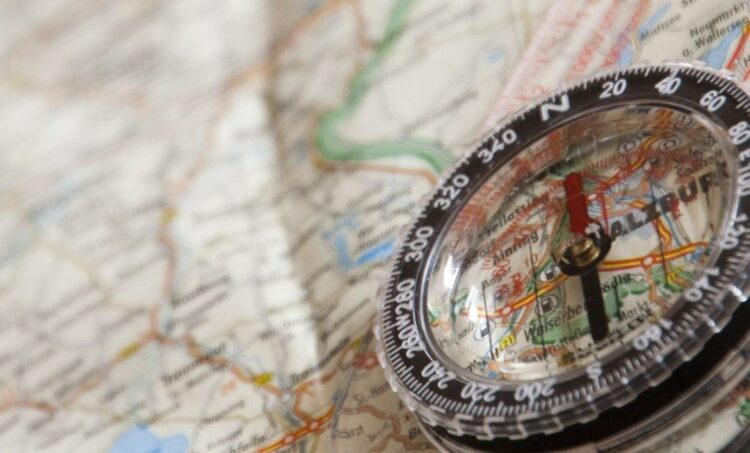# Basic instructions for reading a trekking map

The map is an accurate two-dimensional representation of an area with a specific ratio (scale). Imagine that you are in the mountains and your expensive cell phone or GPS runs out of battery or loses signal. A humble map and a compass is all you need. So the knowledge of how to read a map and how that helps in orientation is one of the basic “musts” for all those who walk around the mountains.

The four basic elements that describe a map and we need to know in order to read it are the following. North Indication, Scale, Contour lines and Legend.

North Indication on the map

On all maps there is an arrow pointing to the north. It is always at the top of the map, always symbolized by the letter N (North) and in combination with a compass shows the hiker the necessary orientation, so that he can understand where he is and where he wants to go.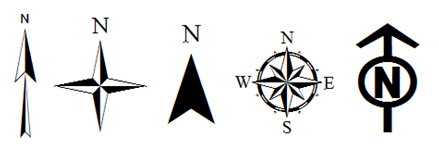Scale

The scale is the ratio between the actual distance and its representation on the map. Based on the scale, the map shows a reduced part of the earth’s surface. The scale is almost always at the bottom of the map. For example a scale 1: 25,000 means that 1cm on the map is equal to 25,000cm on the ground or 250m, a scale of 1: 15,000 means that 1cm is equal to 150m and so on. So we can understand that the smaller the scale, the more detail the map has. Based on the scale we can also calculate the distance.

Let’s take an example: In a map with a scale of 1: 25,000 we want to go from point A to point B which are 20cm away on the map. Every 1cm as we said is 250m therefore 20cm x 250m = 5km. Considering that the average speed for a hiker is 4km per hour, we will need a little more than 1 hour to cover the distance. This is quite useful especially when planning large routes. But beware, this simple calculation of the distance between two points, does not take into consideration the terrain, since the map has two dimensions and not three like the real world, neither the maneuvers of the path. If our route is in a flat area, the measurement is close to reality. If not, it’s a good indication to time-plan your course. A good practice to calculate the maneuvers of the route is to place a string on the trail on the map. Then stretch it, measure it and do the math based on the scale. To calculate also the terrain, you must use the contour lines.

Contour lines

The contour lines are the curved lines that we observe on the map and the give very important information about its topography and have the following characteristics

A. They are always closed curved lines.

B. Each line describes points located at the same altitude

C. They are almost parallel to each other and almost never intersect unless they are representing very sharp sections of the surface.

D. Their distance is determined by the inclination of the terrain.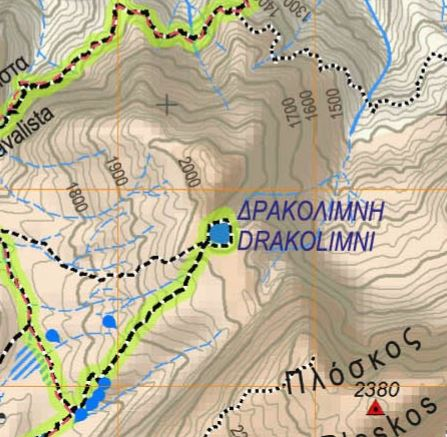In some contour lines there is a number. This number indicates the altitude of the points on this line. For better readability, the altitude does not appear on every line but only in lines that have 100m of altitude difference. Between the altitude reference contour lines there are other lines with a fainter color and without a number. The difference between two sequential lines indicates the difference in altitude, it is called equivalence and it is mentioned in the legend of each map. Since the equivalence is constant, you realize that the denser the contour lines are the higher the inclination. By understanding the meaning of equivalence, we can read and imagine the map in 3 dimensions and this is helping us to calculate our hike with relative accuracy. See some examples below: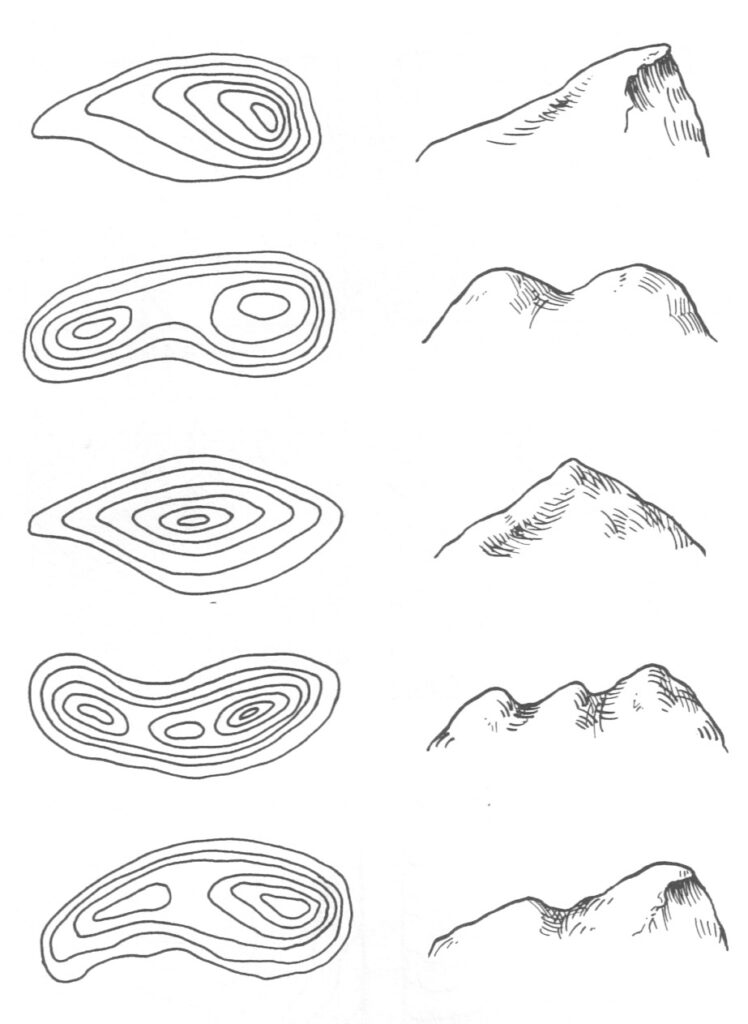Using the scale of the map we measured the distance in 2 dimensions (horizontal distance). Using the contour lines, we can now calculate the actual distance of our route. To do this we must use school geometry and the Pythagorean Theorem. From the figure we understand that the real distance is described by the relation Slope distance = square root [(altitude difference)2 + (horizontal distance)2]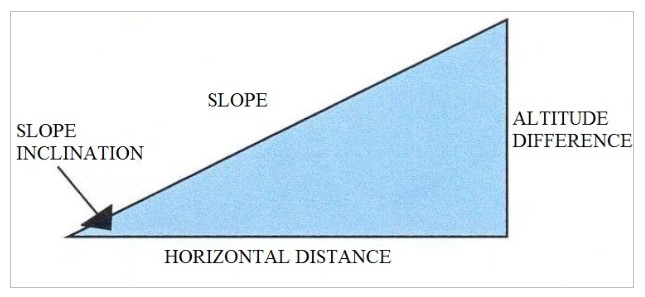But because we do not have a pocket computer on the mountain, we found in an old scout manual a very useful table that helps to calculate the extra distance and the inclination of the slope.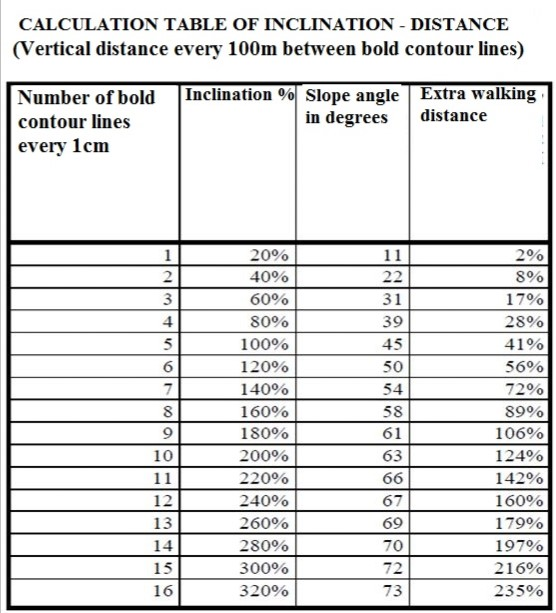Legend

The legend is the dictionary for reading the map. Decrypts the map, and provides explanations for all the symbols on the map. The types of symbols that can be found in the legend of a hiking map are symbols depicting settlements, peaks, springs, caves, paths, rivers, etc. Also in the legend of a map the scale and the equivalence of the contour lines, are mentioned.

Try to practice reading the map of a known area. Imagine the ground and how it is related to contour lines. In the field, select features such as peaks, cliffs and ridges and study them on the map. Practice often and in a short time you will master one of the most basic mountaineering skills.